Documentation

# comm.SymbolSynchronizer

Correct symbol timing clock skew

## Description

The `SymbolSynchronizer` System object™ corrects for the clock skew between a single-carrier transmitter and receiver.

To correct for symbol timing clock skew:

1. Define and set up the `SymbolSynchronizer` object. See Construction.

2. Call `step` to correct for the clock skew between a transmitter and receiver according to the properties of `comm.SymbolSynchronizer`. The length of the output vector varies depending on the timing error and the synchronizer properties. The behavior of `step` is specific to each object in the toolbox.

The listed characteristics apply to the symbol synchronizer:

• PAM, PSK, and QAM modulation schemes are supported.

• The input operates on a sample rate basis, while the output signal operates on a symbol rate basis.

• The damping factor, normalized loop bandwidth, and detector gain properties are tunable so that they can be easily adjusted to improve synchronizer performance.

### Note

Starting in R2016b, instead of using the `step` method to perform the operation defined by the System object™, you can call the object with arguments, as if it were a function. For example, ```y = step(obj,x)``` and `y = obj(x)` perform equivalent operations.

## Construction

`S = comm.SymbolSynchronizer` creates a symbol synchronizer System object, `S`, that corrects for the clock skew between a single-carrier transmitter and receiver.

`S = comm.SymbolSynchronizer(Name,Value)` creates a symbol synchronizer object, `S`, with each specified property `Name` set to the specified `Value`. You can specify additional name-value pair arguments in any order as (`Name1`,`Value1`,...,`NameN`,`ValueN`).

## Properties

`Modulation`

Modulation type

Specify the modulation type as `'PAM/PSK/QAM'`, or `'OQPSK'`. The default value is `'PAM/PSK/QAM'`. This property is nontunable.

`TimingErrorDetector`

Timing error detector

Specify the timing error detection method as ```Zero-Crossing (decision-directed)```, `Gardner (non-data-aided)`, ```Early-Late (non-data-aided)```, or `Mueller-Muller (decision-directed)`. The selection controls the timing error detection scheme used in the synchronizer. The default value is `Zero-Crossing ( decision-directed)`. This property is nontunable.

`SamplesPerSymbol`

Samples per symbol

Specify the number of samples per symbol, s, as a real positive finite integer scalar, such that s ≥ 2. The default value is `2`. This property is nontunable.

`DampingFactor`

Damping factor of the loop filter

Specify the damping factor of the loop filter as a positive scalar. The default value is `1`. This property is tunable.

`NormalizedLoopBandwidth`

Normalized bandwidth of the loop filter

Specify the normalized loop bandwidth as a real scalar having a value between 0 and 1. The loop bandwidth is normalized by the sample rate of the input signal. The default value is `0.01`. This property is tunable.

### Note

It is recommended to set `NormalizedLoopBandwidth` to less than `0.1` to ensure that the symbol synchronizer locks.

`DetectorGain`

Gain of the phase detector

Specify the detector gain as a real positive finite scalar. The default value is `2.7`. This property is tunable.

## Methods

 reset Reset states of the symbol synchronizer object step Correct symbol timing clock skew
Common to All System Objects
`release`

Allow System object property value changes

## Examples

expand all

Correct for a fixed symbol timing error on a noisy QPSK signal.

Create raised cosine transmit and receive filter System objects™.

```txfilter = comm.RaisedCosineTransmitFilter( ... 'OutputSamplesPerSymbol',4); rxfilter = comm.RaisedCosineReceiveFilter( ... 'InputSamplesPerSymbol',4, ... 'DecimationFactor',2);```

Create a delay objects to introduce a fixed timing error of 2 samples(which is equivalent to 1/2 symbol), and to account for the system delay due to the RRC filters and the fixed delay.

```fixedDelay = dsp.Delay(2); filterDelay = dsp.Delay(txfilter.FilterSpanInSymbols/2 + ... rxfilter.FilterSpanInSymbols/2 + 1);```

Create a `SymbolSynchronizer` object to eliminate the timing error.

`symbolSync = comm.SymbolSynchronizer;`

Generate random 4-ary symbols and apply QPSK modulation.

```data = randi([0 3],5000,1); modSig = pskmod(data,4,pi/4);```

Filter the modulated signal through a raised cosine transmit filter and apply a fixed delay.

```txSig = txfilter(modSig); delaySig = fixedDelay(txSig);```

Pass the delayed signal through an AWGN channel with a 15 dB signal-to-noise ratio.

`rxSig = awgn(delaySig,15,'measured');`

Filter the received signal and display its scatter plot. The scatter plot shows that the received signal does not align with the expected QPSK reference constellation due to the timing error.

```rxSample = rxfilter(rxSig); scatterplot(rxSample(1001:end),2)```Correct for the symbol timing error by using the symbol synchronizer object. Display the scatter plot and observe that the synchronized signal now aligns with the expected QPSK constellation.

```rxSync = symbolSync(rxSample); scatterplot(rxSync(1001:end),2)```Decode the QPSK signal and display number of errors.

`recData = pskdemod(rxSync,4,pi/4);`

Account for system delay and generate a BER.

`[numErr,ber] = biterr(data(1:end-filterDelay.Length),recData(filterDelay.Length+1:end))`
```numErr = 0 ```
```ber = 0 ```

Correct symbol timing error in a PSK67 transmission signal. Check BER of synchronized received signal.

Create root raised cosine (RRC) transmit and receive filter System objects.

```samplesPerSymbol = 4; txfilter = comm.RaisedCosineTransmitFilter(... 'OutputSamplesPerSymbol',samplesPerSymbol); rxfilter = comm.RaisedCosineReceiveFilter(... 'InputSamplesPerSymbol',samplesPerSymbol,'DecimationFactor',1);```

Create a delay objects to introduce a fixed timing error of 2 samples, and to account for the total system delay due the RRC filters and the fixed delay.

```fixedDelay = dsp.Delay(2); filterDelay = dsp.Delay(txfilter.FilterSpanInSymbols/2 + ... rxfilter.FilterSpanInSymbols/2 + fixedDelay.Length/2);```

Create a SymbolSynchronizer object to eliminate the timing error.

```symbolSync = comm.SymbolSynchronizer(... 'SamplesPerSymbol',samplesPerSymbol, ... 'NormalizedLoopBandwidth',0.01, ... 'DampingFactor',1.0, ... 'TimingErrorDetector','Early-Late (non-data-aided)');```

Generate random data symbols and apply bpsk modulation.

```data = randi([0 1],20000,1); modSig = pskmod(data,2);```

Filter the modulated signal through the transmit RRC filter and apply the fixed delay to the signal.

```txSig = txfilter(modSig); delayedSig = fixedDelay(txSig);```

Pass the delayed signal through an AWGN channel with a 15 dB signal-to-noise ratio.

`rxSig = awgn(delayedSig,15,'measured');`

RRC filter the received signal and display its scatter plot. Because of the timing error, the received signal does not align with the expected BPSK reference constellation.

```rxSample = rxfilter(rxSig); scatterplot(rxSample(10000:end),2)```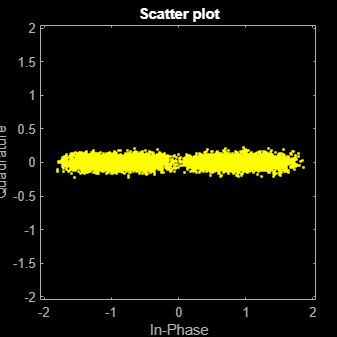Use the symbol synchronizer object to correct the symbol timing error. Redisplay the scatter plot. The synchronized signal now aligns with the expected psk67 constellation.

```rxSync = symbolSync(rxSample); scatterplot(rxSync(10000:end),2)```Decode the BPSK signal.

`rxCoded = pskdemod(rxSync,2);`

Account for system delay and calculate the BER.

`[numErr,ber] = biterr(filterDelay(data),rxCoded)`
```numErr = 8 ```
```ber = 4.0000e-04 ```

Recover from symbol timing and frequency offset errors by using the `comm.CarrierSynchronizer` and `comm.SymbolSynchronizer` objects.

Configure Example

Specify the samples per symbol parameter. Create a matched pair of raised cosine filter objects.

```sps = 8; txfilter = comm.RaisedCosineTransmitFilter('FilterSpanInSymbols',10, ... 'OutputSamplesPerSymbol',sps,'Gain',sqrt(sps)); rxfilter = comm.RaisedCosineReceiveFilter('FilterSpanInSymbols',10, ... 'InputSamplesPerSymbol',sps,'DecimationFactor',sps/2,'Gain',sqrt(1/sps)); ```

Create a `PhaseFrequencyOffset` object to introduce a 100 Hz Doppler shift.

```doppler = comm.PhaseFrequencyOffset('FrequencyOffset',100, ... 'PhaseOffset',45,'SampleRate',1e6); ```

Create a variable delay object to introduce timing offsets.

```varDelay = dsp.VariableFractionalDelay; ```

Create carrier and symbol synchronizer objects to correct for a Doppler shift and a timing offset, respectively.

```carrierSync = comm.CarrierSynchronizer('SamplesPerSymbol',2); symbolSync = comm.SymbolSynchronizer(... 'TimingErrorDetector','Early-Late (non-data-aided)', ... 'SamplesPerSymbol',2); ```

Create constellation diagram objects to view the results.

```refConst = qammod(0:15,16,'UnitAveragePower',true); cdReceive = comm.ConstellationDiagram('ReferenceConstellation',refConst, ... 'SamplesPerSymbol',sps,'Title','Received Signal'); cdDoppler = comm.ConstellationDiagram('ReferenceConstellation',refConst, ... 'SamplesPerSymbol',2,'Title','Frequency Corrected Signal'); cdTiming = comm.ConstellationDiagram('ReferenceConstellation',refConst, ... 'SamplesPerSymbol',2,'Title','Frequency and Timing Synchronized Signal'); ```

Main Processing Loop

Perform the following operations:

• Generate random symbols and apply QAM modulation.

• Filter the modulated signal.

• Apply frequency and timing offsets.

• Pass the transmitted signal through an AWGN channel.

• Correct for the Doppler shift.

• Filter the received signal.

• Correct for the timing offset.

```for k = 1:15 data = randi([0 15],2000,1); modSig = qammod(data,16,'UnitAveragePower',true); txSig = txfilter(modSig); txDoppler = doppler(txSig); txDelay = varDelay(txDoppler,k/15); rxSig = awgn(txDelay,25); rxFiltSig = rxfilter(rxSig); rxCorr = carrierSync(rxFiltSig); rxData = symbolSync(rxCorr); end ```

Visualization

Plot the constellation diagrams of the received signal, the frequency corrected signal, and the frequency and timing synchronized signal. While specific constellation points cannot be identified in the received signal and only partially identified in the frequency corrected signal, the timing and frequency synchronized signal aligns with the expected QAM constellation points.

```cdReceive(rxSig) ```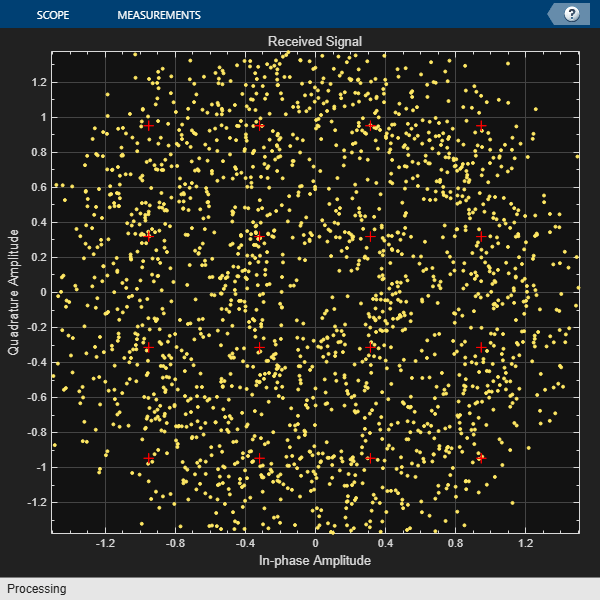```cdDoppler(rxCorr) ``````cdTiming(rxData) ```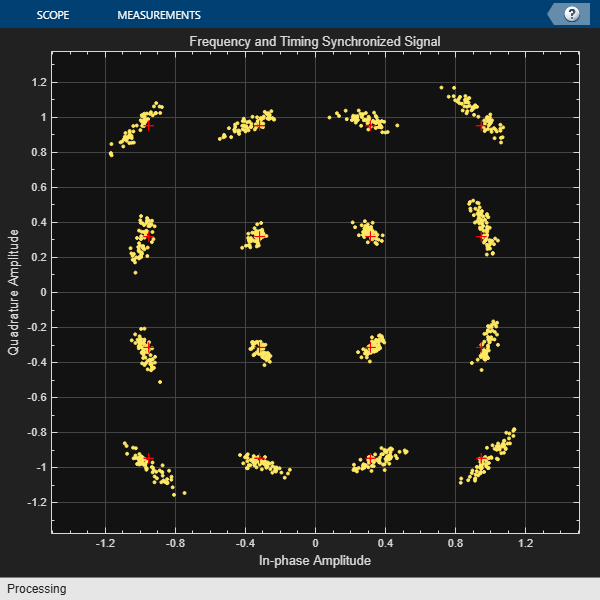Correct for a monotonically increasing symbol timing error on a noisy 8-PSK signal and display the normalized timing error.

Set example parameters.

```fsym = 5000; % Symbol rate (Hz) sps = 2; % Samples per symbol fsamp = sps*fsym; % Sample rate (Hz)```

Create raised cosine transmit and receive filter System objects™.

```txfilter = comm.RaisedCosineTransmitFilter( ... 'OutputSamplesPerSymbol',sps); rxfilter = comm.RaisedCosineReceiveFilter( ... 'InputSamplesPerSymbol',sps, ... 'DecimationFactor',1);```

Create a variable fractional delay object to introduce a monotonically increasing timing error.

`varDelay = dsp.VariableFractionalDelay;`

Create a `SymbolSynchronizer` object to eliminate the timing error.

```symbolSync = comm.SymbolSynchronizer(... 'TimingErrorDetector','Mueller-Muller (decision-directed)', ... 'SamplesPerSymbol',sps);```

Generate random 8-ary symbols and apply PSK modulation.

```data = randi([0 7],5000,1); modSig = pskmod(data,8,pi/8);```

Filter the modulated signal through a raised cosine transmit filter and apply a monotonically increasing timing delay.

```vdelay = (0:1/fsamp:1-1/fsamp)'; txSig = txfilter(modSig); delaySig = varDelay(txSig,vdelay);```

Pass the delayed signal through an AWGN channel with a 15 dB signal-to-noise ratio.

`rxSig = awgn(delaySig,15,'measured');`

Filter the received signal and display its scatter plot. The scatter plot shows that the received signal does not align with the expected 8-PSK reference constellation due to the timing error.

```rxSample = rxfilter(rxSig); scatterplot(rxSample,sps)```Correct for the symbol timing error by using the symbol synchronizer. Display the scatter plot and observe that the synchronized signal now aligns with the 8-PSK constellation.

```[rxSym,tError] = symbolSync(rxSample); scatterplot(rxSym(1001:end))```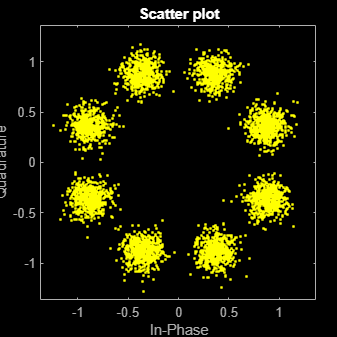Plot the timing error estimate. You can see that the normalized timing error ramps up to 1 sample.

```figure plot(vdelay,tError) xlabel('Time (s)') ylabel('Timing Error (samples)')```## Algorithms

### Overview

The symbol timing synchronizer is based on a phased lock loop (PLL) algorithm consisting of four components:

• A timing error detector (TED)

• An interpolator

• An interpolation controller

• A loop filter

• For OQPSK, the in-phase and quadrature signal components are first aligned (as in QPSK) using a buffer (state) to cache the last half symbol of the previous input. After initial alignment, the remaining synchronization processing is QPSK.

A generalized diagram of a timing synchronizer is shown below.In the figure, x(t) is the received sample signal after the matched filter and $x\left(k{T}_{s}+\stackrel{^}{\tau }\right)$ is the symbol signal corrected for the clock skew between the transmitter and receiver.

### Timing Error Detection

The `SymbolSynchronizer` object supports four types of timing error detection: Zero-Crossing, Gardner, Early-Late, and Mueller-Muller.

A non-data-aided timing error detector (TED) uses received samples without any knowledge of the transmitted signals and performing any estimation.

A decision-directed TED uses sign function to estimate the in-phase and quadrature components of received samples.

The `Zero-Crossing (decision-directed)` and ```Mueller-Muller (decision-directed)``` methods estimate the timing error based on the sign of the in-phase and quadrature components of signals passed to the synchronizer. As a result, the decision-directed methods are not recommended for constellations that have points with either a zero in-phase or quadrature component. For example, QPSK modulation with a zero phase offset having points at {`1+0i`, `0+1i`, `-1+0i`, and `0−1i`} would not be suitable for these methods.

In the table, $x\left(k{T}_{s}+\stackrel{^}{\tau }\right)$ and $y\left(k{T}_{s}+\stackrel{^}{\tau }\right)$ are the in-phase and quadrature components of the signals input to the timing error detector, where $\stackrel{^}{\tau }$ is the estimated timing error. The coefficients ${\stackrel{^}{a}}_{0}\left(k\right)$ and ${\stackrel{^}{a}}_{1}\left(k\right)$ are the estimates of $x\left(k{T}_{s}+\stackrel{^}{\tau }\right)$ and $y\left(k{T}_{s}+\stackrel{^}{\tau }\right)$. These estimates are made by applying the `sign` function to the in-phase and quadrature components and are used only for the decision-directed TED modes.

TED TypeExpression
Zero-Crossing$e\left(k\right)=x\left(\left(k-1/2\right){T}_{s}+\stackrel{^}{\tau }\right)\left[{\stackrel{^}{a}}_{0}\left(k-1\right)-{\stackrel{^}{a}}_{0}\left(k\right)\right]+y\left(\left(k-1/2\right){T}_{s}+\stackrel{^}{\tau }\right)\left[{\stackrel{^}{a}}_{1}\left(k-1\right)-{\stackrel{^}{a}}_{1}\left(k\right)\right]$
Gardner$e\left(k\right)=x\left(\left(k-1/2\right){T}_{s}+\stackrel{^}{\tau }\right)\left[x\left(\left(k-1\right){T}_{s}+\stackrel{^}{\tau }\right)-x\left(k{T}_{s}+\stackrel{^}{\tau }\right)\right]+y\left(\left(k-1/2\right){T}_{s}+\stackrel{^}{\tau }\right)\left[y\left(\left(k-1\right){T}_{s}+\stackrel{^}{\tau }\right)-y\left(k{T}_{s}+\stackrel{^}{\tau }\right)\right]$
Early-Late$e\left(k\right)=x\left(k{T}_{s}+\stackrel{^}{\tau }\right)\left[x\left(\left(k+1/2\right){T}_{s}+\stackrel{^}{\tau }\right)-x\left(\left(k-1/2\right){T}_{s}+\stackrel{^}{\tau }\right)\right]+y\left(k{T}_{s}+\stackrel{^}{\tau }\right)\left[y\left(\left(k+1/2\right){T}_{s}+\stackrel{^}{\tau }\right)-y\left(\left(k-1/2\right){T}_{s}+\stackrel{^}{\tau }\right)\right]$
Mueller-Muller$e\left(k\right)={\stackrel{^}{a}}_{0}\left(k-1\right)x\left(k{T}_{s}+\stackrel{^}{\tau }\right)-{\stackrel{^}{a}}_{0}\left(k\right)x\left(\left(k-1\right){T}_{s}+\stackrel{^}{\tau }\right)+{\stackrel{^}{a}}_{1}\left(k-1\right)y\left(k{T}_{s}+\stackrel{^}{\tau }\right)-{\stackrel{^}{a}}_{1}\left(k\right)y\left(\left(k-1\right){T}_{s}+\stackrel{^}{\tau }\right)$

#### Zero-Crossing Method

The Zero-Crossing method is a decision-directed technique that requires two samples per symbol at the synchronizer’s input. It performs well in low SNR conditions for all values of excess bandwidth, and in moderate SNR conditions for moderate excess bandwidth factors (~0.4 - ~0.6).

#### Gardner Method

The Gardner method is a non-data-aided feedback method that is independent of carrier phase recovery. It is suitable for both baseband systems and modulated carrier systems. More specifically, this method is suitable for systems that use a linear modulation type with Nyquist pulses that have an excess bandwidth between approximately 40% and 100%. Examples of suitable systems are those that use pulse amplitude modulation (PAM), phase shift keying (PSK) modulation, or quadrature amplitude modulation (QAM), and that shape the signal using raised cosine filters whose rolloff factor is between 0.4 and 1. In the presence of noise, the performance of this timing recovery method improves as the excess bandwidth (rolloff factor in the case of a raised cosine filter) increases.

Gardner's method is similar to the early-late gate method.

#### Early-Late Method

The Early-Late method is also a non-data-aided feedback method. It is suitable for systems that use a linear modulation type, such as pulse amplitude modulation (PAM), phase shift keying (PSK) modulation, or quadrature amplitude modulation (QAM), with Nyquist pulses (for example, using a raised cosine filter). In the presence of noise, the performance of this timing recovery method improves as the pulse's excess bandwidth (rolloff factor in the case of a raised cosine filter) increases.

The Early-Late method is similar to the Gardner method. Compared to the Gardner method, the Early-Late method has higher self noise and thus does not perform as well as the Gardner method in systems with high SNR values.

#### Mueller-Muller Method

The Mueller-Muller method is a decision-directed feedback method that requires prior recovery of the carrier phase.

When the input signal has Nyquist pulses (for example, using a raised cosine filter), this method has no self noise. In the presence of noise, the performance of the Mueller-Muller method improves as the pulse's excess bandwidth factor decreases, making the method a good candidate for narrowband signaling.

### Interpolator

The time delay is estimated from fixed-rate samples of the matched filter, which are asynchronous with the symbol rate. As the resultant samples are not aligned with the symbol boundaries, an interpolator is used to “move” the samples. Because the time delay is unknown, the interpolator must be adaptive. Moreover, because the interpolant is a linear combination of the available samples, it can be thought of as the output of a filter. Consequently, a piecewise parabolic interpolator with a Farrow structure and coefficient α set to 1/2 as described in  is used by the System object.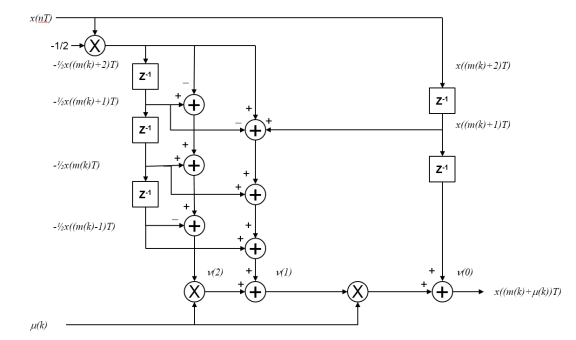### Interpolation Control

Interpolation control provides the interpolator with the basepoint index and the fractional interval, where the basepoint index is the sample index nearest to the interpolant and the fractional interval is the ratio of the time between the interpolant and its basepoint index and the interpolation interval.Interpolation is performed for every sample, where a strobe signal is used to determine if the interpolant is output. The object uses a modulo-1 counter interpolation control to provide the strobe and the fractional interval for use with the interpolator.

### Loop Filter

A proportional-plus integrator (PI) loop filter of the form shown below is used. The proportional gain, K1 and the integrator gain, K2, are calculated by

`${K}_{1}=\frac{-4\zeta \theta }{\left(1+2\zeta \theta +{\theta }^{2}\right){K}_{p}}$`

and

`${K}_{2}=\frac{-4{\theta }^{2}}{\left(1+2\zeta \theta +{\theta }^{2}\right){K}_{p}}\text{\hspace{0.17em}}.$`

The interim term, θ, is given by

`$\theta =\frac{\frac{{B}_{n}{T}_{s}}{N}}{\zeta +\frac{1}{4\zeta }}\text{\hspace{0.17em}},$`

where N, ζ, BnTs, and Kp correspond to the `SamplesPerSymbol`, `DampingFactor`, `NormalizedLoopBandwidth`, and `DetectorGain` properties, respectively.## Selected Bibliography

 Rice, Michael. Digital Communications: A Discrete-Time Approach. Upper Saddle River, NJ: Prentice Hall, 2009, pp. 434–513.

 Mengali, Umberto and Aldo N. D’Andrea. Synchronization Techniques for Digital Receivers. New York: Plenum Press, 1997.